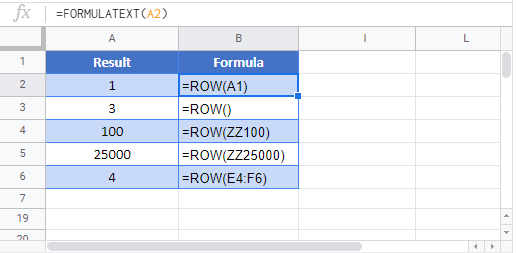# ROW Function Examples – Excel & Google Sheets

This Tutorial demonstrates how to use the Excel ROW Function in Excel to look up the row number.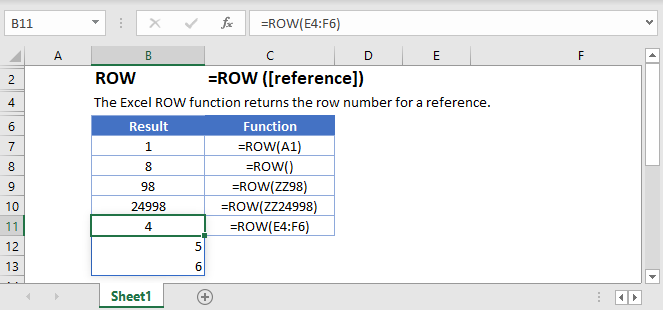## ROW Function Overview

The ROW Function Returns the row number of a cell reference.

To use the ROW Excel Worksheet Function, select a cell and type: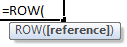(Notice how the formula inputs appear)

## ROW function Syntax and inputs:

reference – Cell reference that you want to determine the row # of.

## ROW Function – Single Cell

The ROW Function returns the row number of a specific cell reference.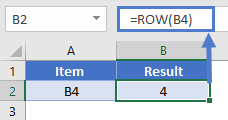## ROW Function with no Reference

If no cell reference is provided, the ROW Function will return the row number where the formula is entered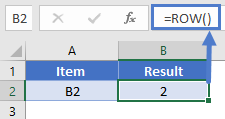## ROW Function with a Range

You can also enter entire ranges of cells into the ROW Function. When doing so, the ROW Function behaves differently in Excel 2019 (or earlier) vs. Excel 365 or newer version of Excel.

## Excel 2019 or older

In previous versions of Excel, the ROW Function returns an array containing the row values of all the cells in the range, but only displays the first result in the cell.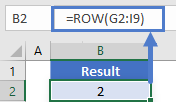If you click the cell containing the formula and press F9, all the results are displayed in curly brackets as an array.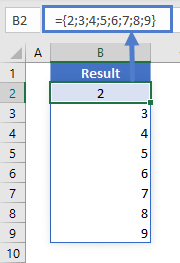## Excel 365

However, Excel 365 (and newer versions of Excel, presumably) comes with a spill range feature. Here, the ROW Function will return the rows of all cells in the range, “spilled” into the next cells.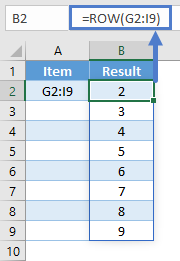## ROW Function in Google Sheets

The ROW Function works exactly the same in Google Sheets as in Excel: